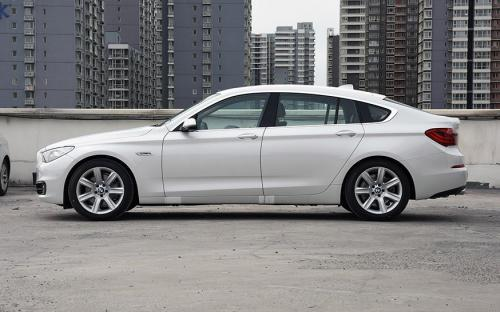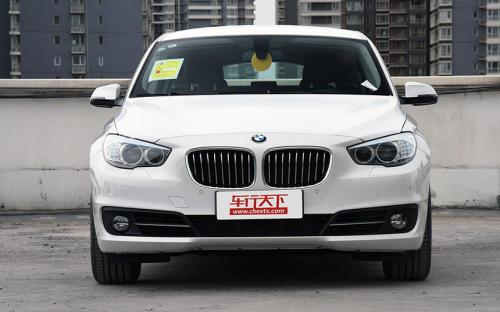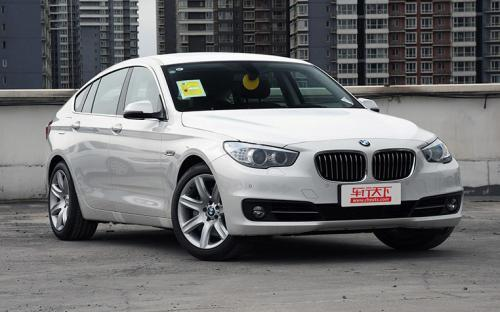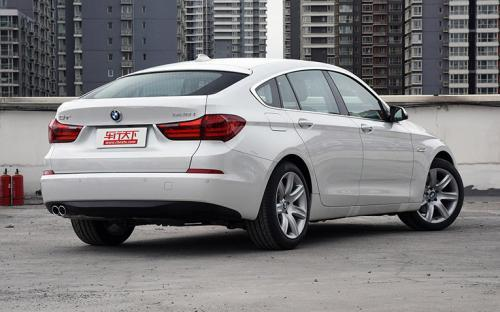宝马(进口) 宝马5系GT2017款最低售价：70.10 万元起

5012(mm)1901(mm)1575(mm)配置亮点：
• 胎压监测装置

• ISOFIX儿童座椅接口

• 车身稳定控制(ESC/ESP/DSC等)

• 电动天窗

• 定速巡航

• 后倒车雷达

• 真皮座椅

• GPS导航系统

• 氙气大灯

• 后视镜加热

• 提交
2017款 528i 领先型 (131张)

宝马(进口) 宝马5系GT 绕车实拍• 宝马(进口) 宝马5系GT 在售车型

排量 车型 厂商指导价 本地最低报价 购车工具
T
528i 领先型 8挡手自一体
70.10万
70.10万

宝马(进口) 宝马5系GT 经销商

查看更多 >>

宝马(进口) 宝马5系GT 动力加速

宝马5系GT 0-100公里加速时间分布在 7.0-7秒 属于 高性能级

动力级别 加速时间 车型
高性能级(1款)
7.0s
528i 领先型

宝马(进口) 宝马5系GT 视频

宝马(进口) 宝马5系GT 新闻资讯

功率679马力 宝马5系GT或推混动车型

进口新车 超过4469次关注

近日，宝马在5系GT上验证了全新的混动系统，未来宝马很有可能会推出量产版5系GT混动车型。若实现量产，新车将会在2020年之前投入市场。

越界时尚范儿 试驾体验宝马5系GT

评测 超过4038次关注

夜深了，霓虹却不肯散去，它们在宝马GT身上留下一层暧昧的光，闪耀不定。今夜不想回家，我和GT毫无目地的游走在这个城市的边缘，不时穿过大片璀璨的灯火和深不可测...

掀背式后备箱三厢车导购--宝马5系GT

专业导购 超过4832次关注

继令人惊异的宝马X6取得意想不到的成功之后，宝马此次将大家的注意力转移到了全新一代的5系车型上，而且在三厢车型之前，率先推出了结合轿车和跑车概念的5系GT（Gra...

Hartge推出宝马5系Gran Turismo改装套件

改装 超过3738次关注

宝马5系GranTurismo刚刚推出不久，改装界已经开始摩拳擦掌准备大干一场了。近日，德国专攻宝马车系的改装厂Hartge率先推出了针对宝马5系GT全系车型的改装部件，其开...

宝马5系GT少量现车 最高优惠17万元

车系文章 超过2460次关注

近日，编辑从位于成都市成双大道中段968号的成都祺宝汽车销售服务有限公司了解到，目前到店购宝马5系GT可享最高17万现金优惠，店内有少量现车。

宝马5系GT赠全额购置税和全车保险

车系文章 超过2862次关注

近日，编辑从宝马品牌官方授权经销商天津市中顺津宝5S店了解到，目前店内宝马5系GT车型少量现车有售，购车赠全额购置税与全车保险，感兴趣的网友可到店内试驾，详情...

宝马5系GT可优惠9.5万元 店内现车销售

车系文章 超过2013次关注

近日，编辑走访经销商东莞市骅宝汽车销售服务有限公司获悉，宝马5系GT可优惠现金9.5万元，店内现车销售。对该车感兴趣的朋友，不妨致电经销商详询。

猜你喜欢

﻿
• 快速找车
• 选择品牌
• 选择品牌
• A  奥迪
• A  阿斯顿·马丁
• A  阿尔法·罗密欧
• B  宝沃
• B  布加迪
• B  巴博斯
• B  保时捷
• B  宾利
• B  奔驰
• B  宝马
• B  本田
• B  别克
• B  标致
• B  比亚迪
• B  宝骏
• B  北汽制造
• B  北汽新能源
• B  北汽幻速
• B  北汽威旺
• B  北京汽车
• B  奔腾
• B  北汽绅宝
• C  长安
• C  长安商用
• C  长城
• C  昌河
• D  大众
• D  道奇
• D  DS
• D  东南
• D  东风风神
• D  东风风行
• D  东风小康
• D  东风风度
• D  东风
• F  福特
• F  丰田
• F  菲亚特
• F  法拉利
• F  福田
• F  福迪
• F  福汽启腾
• G  观致
• G  广汽传祺
• G  广汽吉奥
• G  GMC
• H  红旗
• H  汉腾汽车
• H  哈弗
• H  哈飞
• H  海格
• H  海马
• H  华颂
• H  黄海
• H  华泰
• H  恒天
• J  吉利汽车
• J  捷豹
• J  Jeep
• J  江淮
• J  江铃
• J  金杯
• J  九龙
• J  金旅
• K  凯翼
• K  凯迪拉克
• K  克莱斯勒
• K  科尼塞克
• K  卡威
• K  开瑞
• L  路虎
• L  林肯
• L  劳斯莱斯
• L  兰博基尼
• L  雷克萨斯
• L  铃木
• L  雷诺
• L  理念
• L  力帆
• L  莲花汽车
• L  猎豹
• L  路特斯
• L  陆风
• M  马自达
• M  MG
• M  MINI
• M  玛莎拉蒂
• M  摩根
• M  迈凯轮
• N  纳智捷
• O  欧宝
• O  讴歌
• O  欧朗
• Q  奇瑞
• Q  起亚
• Q  启辰
• R  日产
• R  荣威
• R  瑞麒
• S  三菱
• S  斯威汽车
• S  萨博
• S  smart
• S  斯柯达
• S  斯巴鲁
• S  思铭
• S  双龙
• S  上汽大通
• S  双环
• T  特斯拉
• T  腾势
• W  沃尔沃
• W  五菱汽车
• W  五十铃
• W  威兹曼
• W  威麟
• X  现代
• X  雪佛兰
• X  雪铁龙
• X  西雅特
• Y  一汽
• Y  英菲尼迪
• Y  英致
• Y  依维柯
• Y  野马汽车
• Y  永源
• Z  众泰
• Z  中华
• Z  中兴
• Z  知豆
• 选择车系
• 选择车系
• 车型对比
• 选择品牌
• 选择品牌
• A  奥迪
• A  阿斯顿·马丁
• A  阿尔法·罗密欧
• B  宝沃
• B  布加迪
• B  巴博斯
• B  保时捷
• B  宾利
• B  奔驰
• B  宝马
• B  本田
• B  别克
• B  标致
• B  比亚迪
• B  宝骏
• B  北汽制造
• B  北汽新能源
• B  北汽幻速
• B  北汽威旺
• B  北京汽车
• B  奔腾
• B  北汽绅宝
• C  长安
• C  长安商用
• C  长城
• C  昌河
• D  大众
• D  道奇
• D  DS
• D  东南
• D  东风风神
• D  东风风行
• D  东风小康
• D  东风风度
• D  东风
• F  福特
• F  丰田
• F  菲亚特
• F  法拉利
• F  福田
• F  福迪
• F  福汽启腾
• G  观致
• G  广汽传祺
• G  广汽吉奥
• G  GMC
• H  红旗
• H  汉腾汽车
• H  哈弗
• H  哈飞
• H  海格
• H  海马
• H  华颂
• H  黄海
• H  华泰
• H  恒天
• J  吉利汽车
• J  捷豹
• J  Jeep
• J  江淮
• J  江铃
• J  金杯
• J  九龙
• J  金旅
• K  凯翼
• K  凯迪拉克
• K  克莱斯勒
• K  科尼塞克
• K  卡威
• K  开瑞
• L  路虎
• L  林肯
• L  劳斯莱斯
• L  兰博基尼
• L  雷克萨斯
• L  铃木
• L  雷诺
• L  理念
• L  力帆
• L  莲花汽车
• L  猎豹
• L  路特斯
• L  陆风
• M  马自达
• M  MG
• M  MINI
• M  玛莎拉蒂
• M  摩根
• M  迈凯轮
• N  纳智捷
• O  欧宝
• O  讴歌
• O  欧朗
• Q  奇瑞
• Q  起亚
• Q  启辰
• R  日产
• R  荣威
• R  瑞麒
• S  三菱
• S  斯威汽车
• S  萨博
• S  smart
• S  斯柯达
• S  斯巴鲁
• S  思铭
• S  双龙
• S  上汽大通
• S  双环
• T  特斯拉
• T  腾势
• W  沃尔沃
• W  五菱汽车
• W  五十铃
• W  威兹曼
• W  威麟
• X  现代
• X  雪佛兰
• X  雪铁龙
• X  西雅特
• Y  一汽
• Y  英菲尼迪
• Y  英致
• Y  依维柯
• Y  野马汽车
• Y  永源
• Z  众泰
• Z  中华
• Z  中兴
• Z  知豆
• 选择车系
• 选择车系
• 选择车型
• 选择车型
• 意见反馈